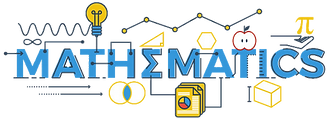13 Number Sense activities designed for older children between grades 2 and 4.

Farm animals jigsaw coordinates. Based on a simple puzzle to recreate a picture of farm animals children use a simple numbered and lettered grid to position the pieces.  Each grid square contains one axis with letters and one axis with numbers.
Number bonds to 100 pizza. There are 6 pizza slices on the board. Each slice needs two pieces of pepperoni.  Both pieces of pepperoni must add up to 100.  The activity changes each time it runs.
Rounding to 10. In this activity children are going to round numbers on the turtle's back to the nearest ten. This activity has been designed so it is easy to change the numbers to suit your children. Content will change positions each time the activity runs.
Skip counting by 10s. Skip Counting Snake 10s. Children practice skip-counting by 10s. (CC.Math.Content.2.NBT.A.2 Count within 1000; skip-count by 5s, 10s, and 100s)
Skip counting by 3s.    3s
Skip counting by 5s.    5s
Skip counting by negative 5s.  -5s

Division snake. Children solve division problems on the rotating snake. In two user mode children could race against each other
Fraction wheels.    Children match the numeric fractions with the equivalent shaded portion of fraction wheels between halves and twelfths. CC: Develop an Understanding of Fractions as Numbers. Understand a fraction 1/b as the quantity formed by 1 part when a whole is partitioned into b equal parts; understand a fraction a/b as the quantity formed by a parts of size 1/b. Content will change every time the activity runs.
Number sequences to 20. Children complete partial sequences of 6 numbers up to 20
Sequencing decimal numbers. Children compare five decimals and order them in ascending values
Money memory game. Children play this classic memory game with cards that have a written sum and cards with the same value in coins.  The card positions change each time the activity runs. Children need to add the sum of the coins and match them with the written sum. Memory Games can help: improve concentration; train visual memory; increase short term memory; increase attention to detail; improve the ability to find similarities and differences in objects; help to classify objects that are grouped by similar traits and improve vocabulary.
Odds and evens Venn. Sorting even numbers from numbers in the 3x table using a Venn diagram.

## Activity House App